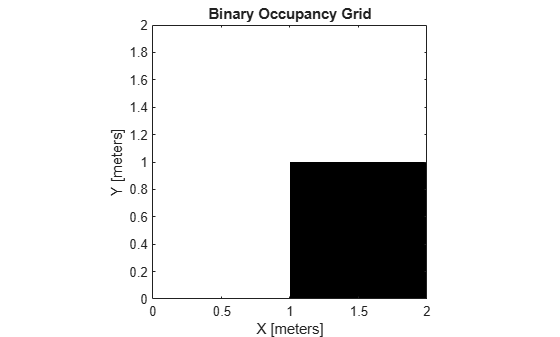Documentation

getOccupancy

Get occupancy value of locations

Description

example

occVal = getOccupancy(map,xy) returns an array of occupancy values at the xy locations in the world frame. Unknown locations, including outside the map, return map.DefaultValue.

example

occVal = getOccupancy(map,xy,"local") returns an array of occupancy values at the xy locations in the local frame.

example

occVal = getOccupancy(map,ij,"grid") specifies ij grid cell indices instead of xy locations.

[occVal,validPts] = getOccupancy(___) additionally outputs an n-element vector of logical values indicating whether input coordinates are within the map limits.

occMatrix = getOccupancy(map) returns all occupancy values in the map as a matrix.

occMatrix = getOccupancy(map,bottomLeft,matSize) returns a matrix of occupancy values by specifying the bottom-left corner location in world coordinates and the matrix size in meters.

occMatrix = getOccupancy(map,bottomLeft,matSize,"local") returns a matrix of occupancy values by specifying the bottom-left corner location in local coordinates and the matrix size in meters.

occMatrix = getOccupancy(map,topLeft,matSize,"grid") returns a matrix of occupancy values by specifying the top-left cell index in grid indices and the matrix size.

Examples

collapse all

Create an empty binary occupancy grid map.

map = binaryOccupancyMap(10,10,20);

Input pose of the vehicle, ranges, angles, and the maximum range of the laser scan.

pose = [5,5,0];
ranges = 3*ones(100,1);
angles = linspace(-pi/2,pi/2,100);
maxrange = 20;

Create a lidarScan object with the specified ranges and angles.

scan = lidarScan(ranges,angles);

Insert the laser scan data into the occupancy map.

insertRay(map,pose,scan,maxrange);

Show the map to see the results of inserting the laser scan.

show(map)Check the occupancy of the spot directly in front of the vehicle.

getOccupancy(map,[8 5])
ans = logical
1

Access occupancy values and check their occupancy status based on the occupied and free thresholds of the occupancyMap object.

Create a matrix and populate it with values. Use this matrix to create an occupancy map.

p = zeros(20,20);
p(11:20,11:20) = ones(10,10);
map = binaryOccupancyMap(p,10);
show(map)Get the occupancy of different locations and check their occupancy statuses. The occupancy status returns 0 for free space and 1 for occupied space. Unknown values return –1.

pocc = getOccupancy(map,[1.5 1]);
occupied = checkOccupancy(map,[1.5 1]);
pocc2 = getOccupancy(map,[5 5],'grid');

Input Arguments

collapse all

Map representation, specified as a binaryOccupancyMap object. This object represents the environment of the vehicle.

Coordinates in the map, specified as an n-by-2 matrix of [x y] pairs, where n is the number of coordinates. Coordinates can be world or local coordinates depending on the syntax.

Data Types: double

Grid locations in the map, specified as an n-by-2 matrix of [i j] pairs, where n is the number of locations. Grid locations are given as [row col].

Data Types: double

Location of bottom left corner of output matrix in world or local coordinates, specified as a two-element vector, [xCoord yCoord]. Location is in world or local coordinates based on syntax.

Data Types: double

Output matrix size, specified as a two-element vector, [xLength yLength] or [gridRow gridCol]. The size is in world coordinates, local coordinates, or grid indices based on syntax.

Data Types: double

Location of top left corner of grid, specified as a two-element vector, [iCoord jCoord].

Data Types: double

Output Arguments

collapse all

Occupancy values, returned as an n-by-1 column vector equal in length to xy or ij. Occupancy values can be obstacle free (0) or occupied (1).

Valid map locations, returned as an n-by-1 column vector equal in length to xy or ij. Locations inside the map return a value of 1. Locations outside the map limits return a value of 0.

Matrix of occupancy values, returned as matrix with size equal to matSize or the size of map.